Author: Oscar Cronquist Article last updated on April 18, 2018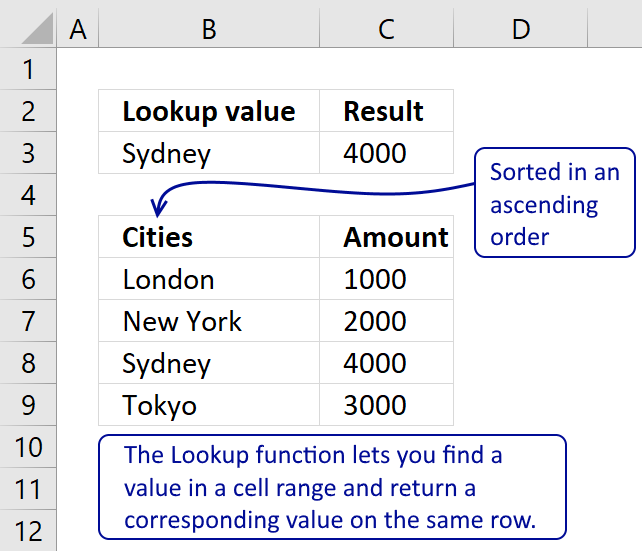The Lookup function lets you find a value in a cell range and return a corresponding value on the same row.

### Excel Function Syntax

LOOKUP(lookup_value, lookup_vector, [result_vector])

### Arguments

 lookup_value Required. The lookup value can be a number, text or a logical value. It can also be a cell reference. This argument can also handle multiple values but you need to enter it as an array function to make it work, there is an example later in this post. lookup_vector Required. This argument must be a single column or single row cell range. result_vector Required. The result vector is optional. If omitted the function returns the lookup value, if found. This argument must be a single column or single row cell range and have the same size as the lookup vector.

The values in lookup_vector must be sorted ascending!

If the LOOKUP function can't find the lookup_value, the function matches the largest value in lookup_vector that is less than or equal to lookup_value.

If lookup_value is smaller than the smallest value in lookup_vector, LOOKUP returns the #N/A error value.

### Be careful with this function

The values in lookup_vector must be sorted ascending but that is not all. You may think you get an error if the lookup value is not found but that is not always the case.

Only if the lookup_value is smaller than the smallest value in lookup_vector, LOOKUP returns #N/A. See cell B4 and C4 in this animated picture.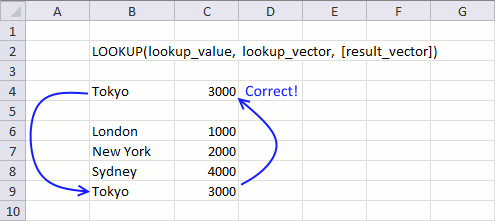So why does "Rome" return 2000? LOOKUP function can't find the lookup_value so it matches the largest value in lookup_vector that is less than or equal to lookup_value.

If Rome would have been in this sorted list, it would be between New York and Sydney. New York is less (alphabetically) than Rome but greater than London. The corresponding value to New York is returned, that value is 2000.

Anchorage is smaller than the smallest value "London" (alphabetically) and Lookup returns #N/A.

You really need to know what you are doing if you are going to use this function. I do recommend using this function with numerical ranges, see next example.

### Find the largest value in lookup_vector that is less than or equal to lookup_value

This example uses a numerical value as a lookup value. If an exact match is not found the closest value is returned as long as it is smaller than the lookup value.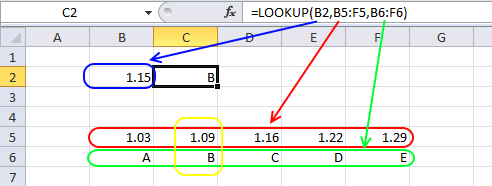Formula in cell C2:

LOOKUP(B2, B5:F5, B6:F6)

returns B.

The largest value that is smaller or equal to 1.15 is 1.09. The corresponding value to 1.09 is B.

### Multiple values

You can concatenate values and search two columns, use the ampersand character "&" to concatenate values in an argument.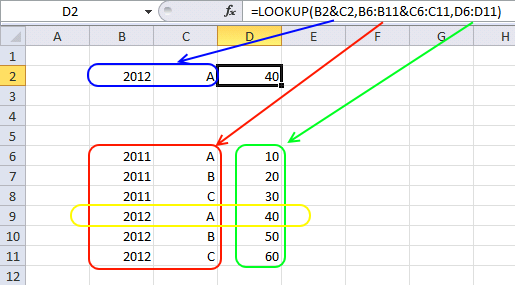Formula in cell D2:

=Lookup(B2&C2, B6:B11&C6:C11, D6:D11)

returns 40.

### Lookups on multiple sheets

This formula allows you to specify a sheet name and a lookup value. Indirect function returns the cell reference depending on the value in cell B3.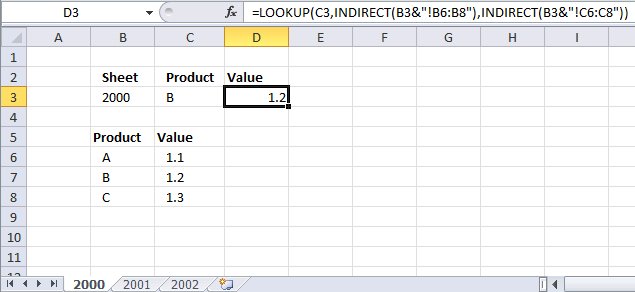Formula in cell D3:

=LOOKUP(C3,INDIRECT(B3&"!B6:B8"),INDIRECT(B3&"!C6:C8"))

### Lookup within a lookup

This formula looks for a value in table1 and uses the corresponding value to do a lookup in table2.

###Formula in cell C3:

=LOOKUP(LOOKUP(B3,B7:B9,C7:C9),E7:E12,F7:F12)

You can see that the Product column exists in both these tables, the tables are in a way related. I have made more formulas for related tables, see these posts:

### Array formula

The first argument lookup_value allows you to enter not only a single value but multiple values. Lookup function returns an array that has the same size as the lookup_value, to demonstrate this I made this formula: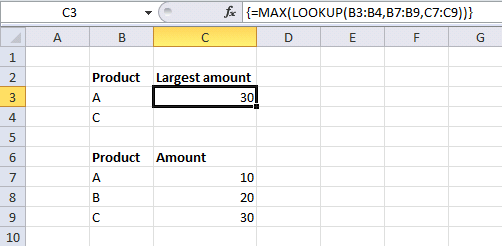Array formula in cell C3:

=MAX(LOOKUP(B3:B4,B7:B9,C7:C9))

How to enter an array formula

1. Select a cell
2. Copy/Paste the formula to  formula bar
3. Press and hold CTRL + SHIFT simultaneously
4. Press Enter

If you did this right the formula begins with a curly bracket { and ends with a curly bracket }. See formula bar in the picture above.

Let me explain this array formula, LOOKUP(B3:B4,B7:B9,C7:C9) becomes LOOKUP({"A";"C"},{"A";"B";"C"},{10;20;30}) and returns this array {10;30}.

MAX function returns the largest value in the array {10;30} , 30 that is.